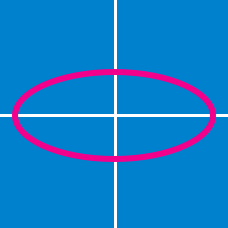Geometry

# Conics - Discriminant

If $$A$$ and $$B$$ are nonzero real numbers, what is the condition on $$A$$ and $$B$$ such that the equation $\frac{x^2}{A^2}+\frac{y^2}{B^2} = 1$ represents an ellipse that is not a circle?

What is the equation of the line passing through the intersection points of the following two curves: ${x}^2+{y}^2-6x-6y+17 = 0 , {x}^2+{y}^2-10x-15y+49= 0?$

What is the area of the set of points in the bounded region ${x}^2 + {y}^2 -8x -8y +28 \leq 0 ?$

If $$5{x}^2+a{y}^2+4x+5y+18=0$$ is an equation that represents a circle, what is the value of $$a ?$$

Which of the following equations represents a different type of conic section than the others?

×